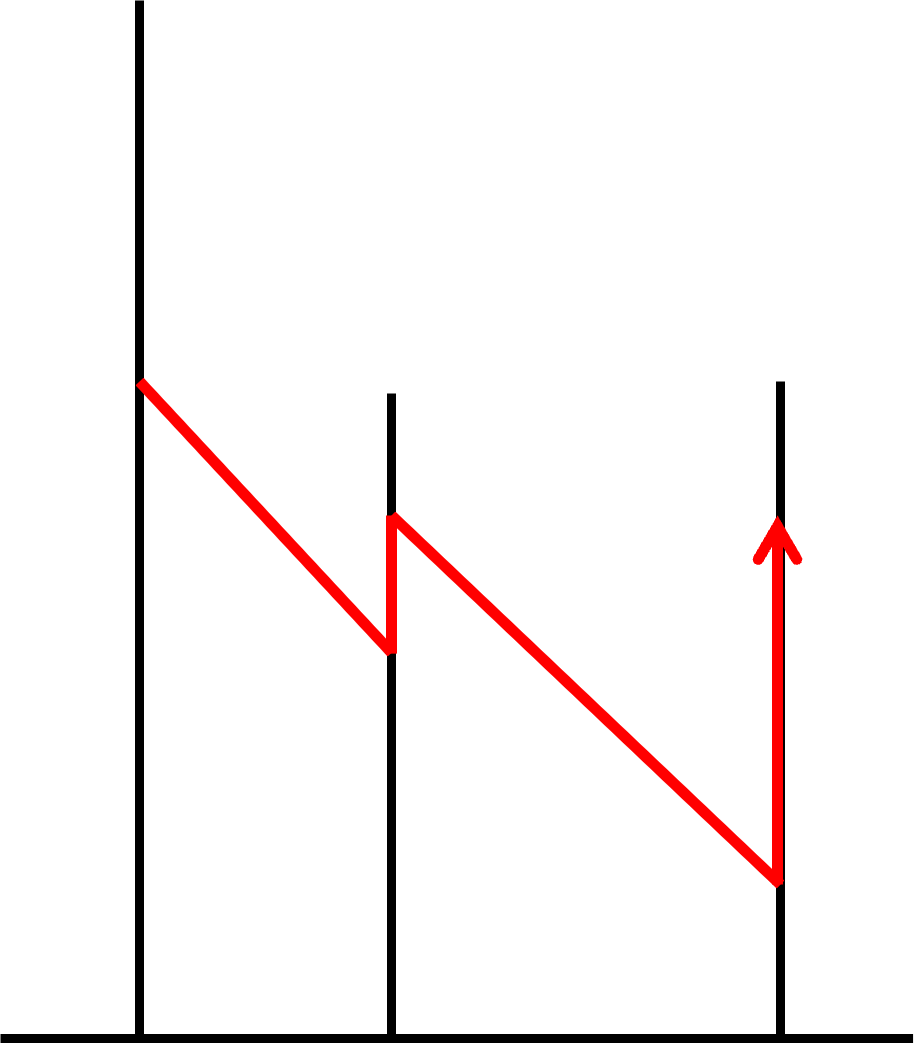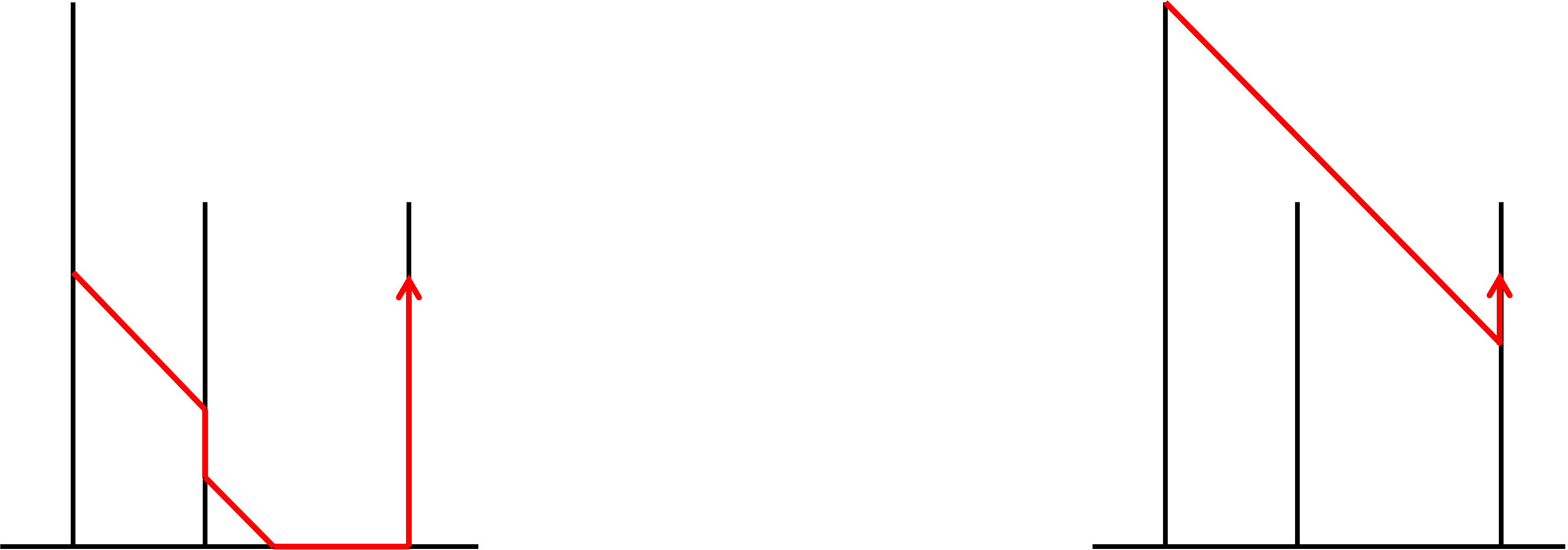### Flying Squirrel

There are $$N$$ upright poles placed in a row, numbered $$1, 2, \ldots, N$$ from left to right. The $$i^{th}$$ pole is affixed to a position $$D_i$$ right of the origin, and extends from the ground to a height $$H_i$$. In addition, pole $$1$$ stands right on the origin, i.e. $$D_1 = 0$$.

A flying squirrel rests at height $$L$$ on pole $$1$$. Now, the squirrel wants to go through all the poles from left to right, and ultimately reach a height $$R$$ on pole $$N$$.

To get between poles, the flying squirrel can glide from one adjacent pole to the next - the squirrel glides in perfect diagonals, dropping a distance $$d$$ in height whenever it glides $$d$$ units towards the right. The squirrel must not land on the ground between poles, but may reach a pole exactly at height $$0$$.

The flying squirrel may also climb up or down each pole. On any pole $$i$$, it takes $$W_i$$ effort for the squirrel to climb $$1$$ unit up the pole, and no effort for it to climb down. The squirrel cannot climb above its current pole's height $$H_i$$, or into the ground.

Figure 1 below shows a valid path the squirrel may take.Figure 1 Figure 2 below shows two invalid paths. The left path is invalid as the flying squirrel lands on the ground between poles; while the right path is invalid as the squirrel does not land on pole $$2$$.Figure 2 Your task is to determine the minimum effort required for the flying squirrel to get to its target position, passing through all poles in between.

### Input format

The first line of input consists of a single integer $$N$$, the number of poles in the arrangement.
$$N$$ lines of input follow. The $$i^{th}$$ of these lines contains 3 integers $$D_i$$, $$H_i$$, and $$W_i$$, denoting that the $$i^{th}$$ pole is located $$D_i$$ from the left, has a height of $$H_i$$, and requires $$W_i$$ effort for the flying squirrel to ascend one unit on.
One line of input follows. This line contains 2 integers $$L_i$$ and $$R_i$$, the initial height of the squirrel on the $$1^{st}$$ pole and its destination height on the $$N^{th}$$ pole respectively.

### Output format

Output a single integer on a single line - the minimum effort required for the squirrel to reach its destination through a valid path, or $$-1$$ if no such path exists.

### Limits

$$2 \leq N \leq 500000$$.
$$0 = D_1 < D_2 < \ldots < D_N \leq 10^9$$.
$$1 \leq H_i \leq 10^9$$ $$(1 \leq i \leq N)$$
$$0 \leq W_i \leq 10^9$$ $$(1 \leq i \leq N)$$
$$0 \leq L \leq H_1$$
$$0 \leq R \leq H_N$$
1 4 $$W_i = 0$$ $$(1 \leq i \leq N)$$
2 13 $$W_i = 1$$ $$(1 \leq i \leq N)$$
3 18 $$W_i \leq W_{i + 1}$$ $$(1 \leq i \leq N - 1)$$
4 15 $$N \leq 500$$
$$H_i \leq 500$$ $$(1 \leq i \leq N)$$
5 18 $$N \leq 5000$$

### Samples

 Sample Input 1 Sample Output 1 3 0 8 3 2 5 4 5 5 6 5 4 18

In this input example, it is optimal for the squirrel to climb 2 units up pole 1 to reach a height of 7, glide to the top of pole 2 and then to height 3 on pole 3, before climbing 2 units up pole 3 to reach its destination.
This takes a total effort of $$2 \cdot 3 + 2 \cdot 6 = 18$$.

### Submitting .cpp to 'Flying Squirrel'

Time Limit: 3 Seconds
Memory Limit: 1024MB
No. of ACs: 5